## 5.359. soft_alldifferent_ctr

Origin
Constraint

$\mathrm{𝚜𝚘𝚏𝚝}_\mathrm{𝚊𝚕𝚕𝚍𝚒𝚏𝚏𝚎𝚛𝚎𝚗𝚝}_\mathrm{𝚌𝚝𝚛}\left(𝙲,\mathrm{𝚅𝙰𝚁𝙸𝙰𝙱𝙻𝙴𝚂}\right)$

Synonyms

$\mathrm{𝚜𝚘𝚏𝚝}_\mathrm{𝚊𝚕𝚕𝚍𝚒𝚏𝚏}_\mathrm{𝚌𝚝𝚛}$, $\mathrm{𝚜𝚘𝚏𝚝}_\mathrm{𝚊𝚕𝚕𝚍𝚒𝚜𝚝𝚒𝚗𝚌𝚝}_\mathrm{𝚌𝚝𝚛}$, $\mathrm{𝚜𝚘𝚏𝚝}_\mathrm{𝚊𝚕𝚕𝚍𝚒𝚏𝚏}_\mathrm{𝚖𝚒𝚗}_\mathrm{𝚌𝚝𝚛}$, $\mathrm{𝚜𝚘𝚏𝚝}_\mathrm{𝚊𝚕𝚕𝚍𝚒𝚏𝚏𝚎𝚛𝚎𝚗𝚝}_\mathrm{𝚖𝚒𝚗}_\mathrm{𝚌𝚝𝚛}$, $\mathrm{𝚜𝚘𝚏𝚝}_\mathrm{𝚊𝚕𝚕𝚍𝚒𝚜𝚝𝚒𝚗𝚌𝚝}_\mathrm{𝚖𝚒𝚗}_\mathrm{𝚌𝚝𝚛}$, $\mathrm{𝚜𝚘𝚏𝚝}_\mathrm{𝚊𝚕𝚕}_\mathrm{𝚎𝚚𝚞𝚊𝚕}_\mathrm{𝚖𝚊𝚡}_\mathrm{𝚌𝚝𝚛}$.

Arguments
 $𝙲$ $\mathrm{𝚍𝚟𝚊𝚛}$ $\mathrm{𝚅𝙰𝚁𝙸𝙰𝙱𝙻𝙴𝚂}$ $\mathrm{𝚌𝚘𝚕𝚕𝚎𝚌𝚝𝚒𝚘𝚗}\left(\mathrm{𝚟𝚊𝚛}-\mathrm{𝚍𝚟𝚊𝚛}\right)$
Restrictions
 $𝙲\ge 0$ $\mathrm{𝚛𝚎𝚚𝚞𝚒𝚛𝚎𝚍}$$\left(\mathrm{𝚅𝙰𝚁𝙸𝙰𝙱𝙻𝙴𝚂},\mathrm{𝚟𝚊𝚛}\right)$
Purpose

Consider the disequality constraints involving two distinct variables $\mathrm{𝚅𝙰𝚁𝙸𝙰𝙱𝙻𝙴𝚂}\left[i\right].\mathrm{𝚟𝚊𝚛}$ and $\mathrm{𝚅𝙰𝚁𝙸𝙰𝙱𝙻𝙴𝚂}\left[j\right].\mathrm{𝚟𝚊𝚛}$ $\left(i of the collection $\mathrm{𝚅𝙰𝚁𝙸𝙰𝙱𝙻𝙴𝚂}$. Among the previous set of constraints, $𝙲$ is greater than or equal to the number of disequality constraints that do not hold.

Example
 $\left(4,〈5,1,9,1,5,5〉\right)$ $\left(1,〈5,1,9,1,2,6〉\right)$ $\left(0,〈5,1,9,0,2,6〉\right)$

Within the collection $〈5,1,9,1,5,5〉$ the first and fifth values, the first and sixth values, the second and fourth values, and the fifth and sixth values are identical. Consequently, the argument $𝙲=4$ is greater than or equal to the number of disequality constraints that do not hold (i.e, 4) and the $\mathrm{𝚜𝚘𝚏𝚝}_\mathrm{𝚊𝚕𝚕𝚍𝚒𝚏𝚏𝚎𝚛𝚎𝚗𝚝}_\mathrm{𝚌𝚝𝚛}$ constraint holds.

Typical
 $𝙲>0$ $𝙲\le |\mathrm{𝚅𝙰𝚁𝙸𝙰𝙱𝙻𝙴𝚂}|*\left(|\mathrm{𝚅𝙰𝚁𝙸𝙰𝙱𝙻𝙴𝚂}|-1\right)/2$ $|\mathrm{𝚅𝙰𝚁𝙸𝙰𝙱𝙻𝙴𝚂}|>1$
Symmetries
• $𝙲$ can be increased.

• Items of $\mathrm{𝚅𝙰𝚁𝙸𝙰𝙱𝙻𝙴𝚂}$ are permutable.

• All occurrences of two distinct values of $\mathrm{𝚅𝙰𝚁𝙸𝙰𝙱𝙻𝙴𝚂}.\mathrm{𝚟𝚊𝚛}$ can be swapped; all occurrences of a value of $\mathrm{𝚅𝙰𝚁𝙸𝙰𝙱𝙻𝙴𝚂}.\mathrm{𝚟𝚊𝚛}$ can be renamed to any unused value.

Arg. properties

Contractible wrt. $\mathrm{𝚅𝙰𝚁𝙸𝙰𝙱𝙻𝙴𝚂}$.

Usage

A soft $\mathrm{𝚊𝚕𝚕𝚍𝚒𝚏𝚏𝚎𝚛𝚎𝚗𝚝}$ constraint.

Remark

The $\mathrm{𝚜𝚘𝚏𝚝}_\mathrm{𝚊𝚕𝚕𝚍𝚒𝚏𝚏𝚎𝚛𝚎𝚗𝚝}_\mathrm{𝚌𝚝𝚛}$ constraint is called $\mathrm{𝚜𝚘𝚏𝚝}_\mathrm{𝚊𝚕𝚕𝚍𝚒𝚏𝚏}_\mathrm{𝚖𝚒𝚗}_\mathrm{𝚌𝚝𝚛}$ or $\mathrm{𝚜𝚘𝚏𝚝}_\mathrm{𝚊𝚕𝚕}_\mathrm{𝚎𝚚𝚞𝚊𝚕}_\mathrm{𝚖𝚊𝚡}_\mathrm{𝚌𝚝𝚛}$ in [HebrardMarxSullivanRazgon09].

Algorithm

Since it focus on the soft aspect of the $\mathrm{𝚊𝚕𝚕𝚍𝚒𝚏𝚏𝚎𝚛𝚎𝚗𝚝}$ constraint, the original article [PetitReginBessiere01] that introduces this constraint describes how to evaluate the minimum value of $𝙲$ and how to prune according to the maximum value of $𝙲$. The corresponding filtering algorithm does not achieve arc-consistency. W.-J. van Hoeve [Hoeve04] presents a new filtering algorithm that achieves arc-consistency. This algorithm is based on a reformulation into a minimum-cost flow problem.

Counting
 Length ($n$) 2 3 4 5 6 7 8 Solutions 15 208 3625 72576 1630279 40632320 1114431777

Number of solutions for $\mathrm{𝚜𝚘𝚏𝚝}_\mathrm{𝚊𝚕𝚕𝚍𝚒𝚏𝚏𝚎𝚛𝚎𝚗𝚝}_\mathrm{𝚌𝚝𝚛}$: domains $0..n$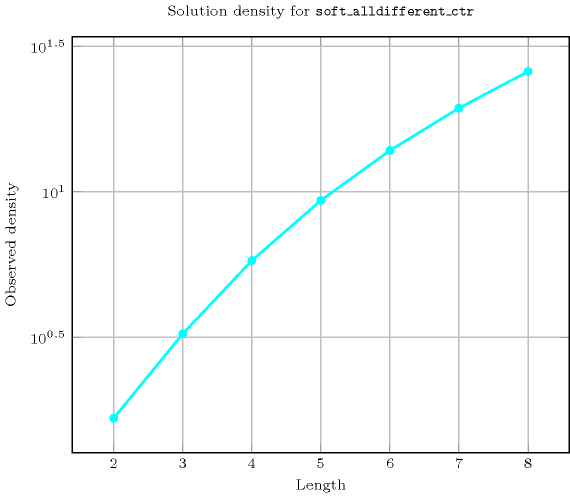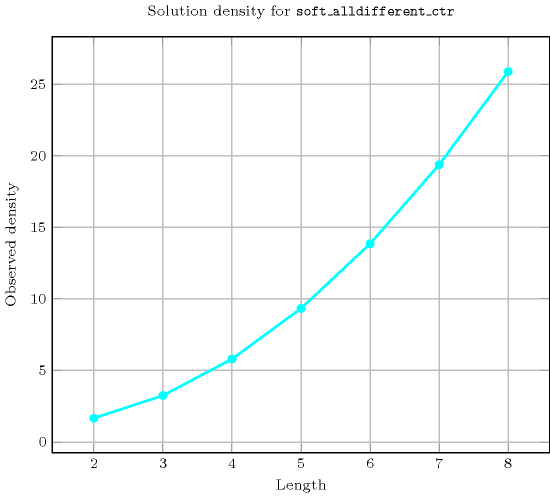Length ($n$)2345678
Total152083625725761630279406323201114431777
 Parameter value

0624120720504040320362880
19604804320428404636805443200
2-60540612080640116928018144000
3-646207320100590158088027881280
4--6207620113190193368036666000
5--6207620113190196896039206160
6--6257770116760205128041111280
7---7770117390208656042522480
8---7770117390208656042628320
9---7770117390208852042769440
10---7776117642209557642938784
11----117642209675243023456
12----117642209675243025976
13----117642209675243030008
14----117642209675243030008
15----117649209714443044120
16-----209714443046136
17-----209714443046136
18-----209714443046136
19-----209714443046136
20-----209714443046136
21-----209715243046712
22------43046712
23------43046712
24------43046712
25------43046712
26------43046712
27------43046712
28------43046721

Solution count for $\mathrm{𝚜𝚘𝚏𝚝}_\mathrm{𝚊𝚕𝚕𝚍𝚒𝚏𝚏𝚎𝚛𝚎𝚗𝚝}_\mathrm{𝚌𝚝𝚛}$: domains $0..n$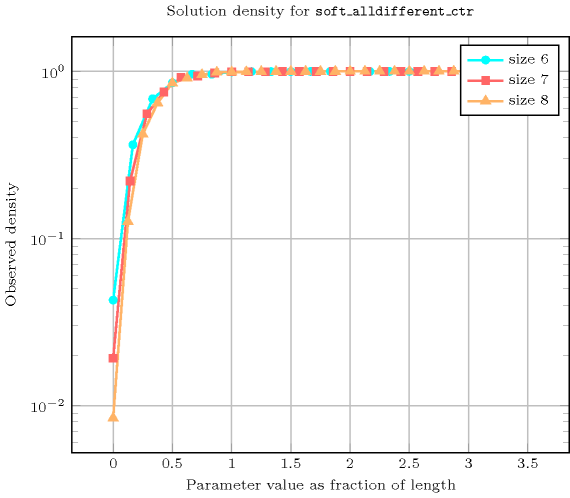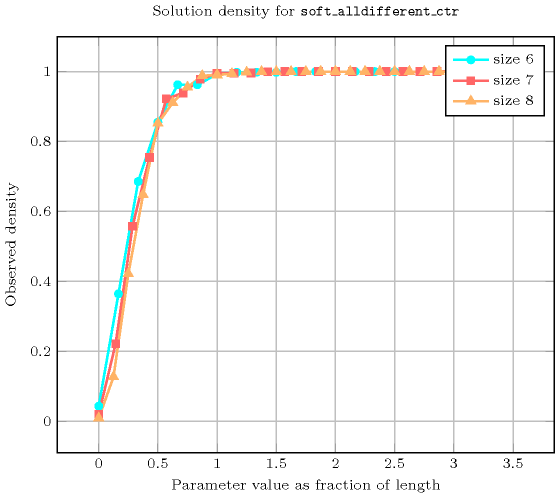Keywords
Arc input(s)

$\mathrm{𝚅𝙰𝚁𝙸𝙰𝙱𝙻𝙴𝚂}$

Arc generator
$\mathrm{𝐶𝐿𝐼𝑄𝑈𝐸}$$\left(<\right)↦\mathrm{𝚌𝚘𝚕𝚕𝚎𝚌𝚝𝚒𝚘𝚗}\left(\mathrm{𝚟𝚊𝚛𝚒𝚊𝚋𝚕𝚎𝚜}\mathtt{1},\mathrm{𝚟𝚊𝚛𝚒𝚊𝚋𝚕𝚎𝚜}\mathtt{2}\right)$

Arc arity
Arc constraint(s)
$\mathrm{𝚟𝚊𝚛𝚒𝚊𝚋𝚕𝚎𝚜}\mathtt{1}.\mathrm{𝚟𝚊𝚛}=\mathrm{𝚟𝚊𝚛𝚒𝚊𝚋𝚕𝚎𝚜}\mathtt{2}.\mathrm{𝚟𝚊𝚛}$
Graph property(ies)
$\mathrm{𝐍𝐀𝐑𝐂}$$\le 𝙲$

Graph model

We generate an initial graph with binary equalities constraints between each vertex and its successors. We use the arc generator $\mathrm{𝐶𝐿𝐼𝑄𝑈𝐸}\left(<\right)$ in order to avoid counting twice the same equality constraint. The graph property states that $𝙲$ is greater than or equal to the number of equalities that hold in the final graph.

Parts (A) and (B) of Figure 5.359.1 respectively show the initial and final graph associated with the Example slot. Since we use the $\mathrm{𝐍𝐀𝐑𝐂}$ graph property, the arcs of the final graph are stressed in bold. Since four equality constraints remain in the final graph the cost variable $𝙲$ is greater than or equal to 4.

##### Figure 5.359.1. Initial and final graph of the $\mathrm{𝚜𝚘𝚏𝚝}_\mathrm{𝚊𝚕𝚕𝚍𝚒𝚏𝚏𝚎𝚛𝚎𝚗𝚝}_\mathrm{𝚌𝚝𝚛}$ constraint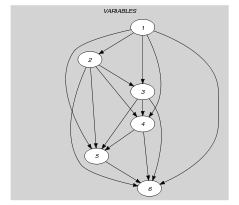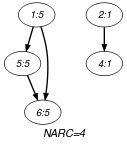(a) (b)